# How to rotate a matrix or vector in Matlab and Octave

To rotate a matrix or vector in Matlab and Octave we use the function rot90().

rot90(M,k)

The first parameter M is the matrix or vector (array)

The second parameter is the number (k) of 90 ° counterclockwise rotations where k is an integer. By default it is k = 1.

Note. In multidimensional arrays, the rot90() function rotates the plane formed by the first and second dimensions.

## Example

Define a vector

V = [1,2,3,4]

This is a row vector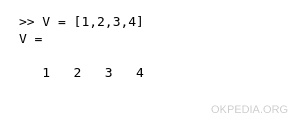The rot90(V) function performs a 90 ° rotation of the vector counterclockwise.

rot90(V)

The output result is a column vector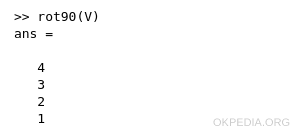To rotate the vector by 180 ° use the rot90() function with the parameter k=2.

rot90(V,2)

The output result is a row vector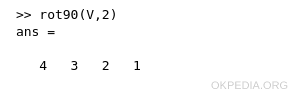Example 2

Define a matrix

M=[[1,2];[3,4]]

It is a square matrix 2x2Rotate the matrix by 90° counterclockwise using the function rot90()

rot90(M)

The output result is the matrix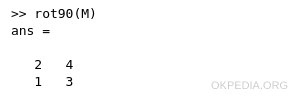To turn it by 180° counterclockwise use rot90() with the parameter k=2

rot90(M,2)

The output result is a rotated matrix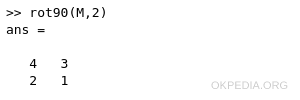To rotate by 270° counterclockwise use rot90() with parameter k=3

rot90(M,3)

The output result is a matrix 2x2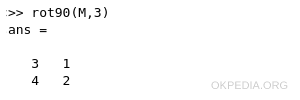https://how.okpedia.org/en/matlab/how-to-rotate-a-matrix-or-vector-in-matlab-and-octaveReport us an error or send a suggestion to improve this page

Matrix in Matlab/Octave